# The Expected Logarithm of a Noncentral Chi-Square Random Variable

In the following lemma a closed form expression is given for the expected value of the logarithm of a noncentral chi-square random variable with an even number of degrees of freedom.

## Lemma

Let the random variable V have a noncentral chi-square distribution with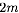degrees of freedom, i.e.,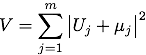where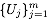are IID circularly-symmetric zero-mean unit-variance complex Gaussians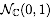and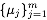are complex constants. Then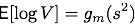where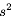denotes the noncentrality parameter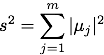and where the functionis defined as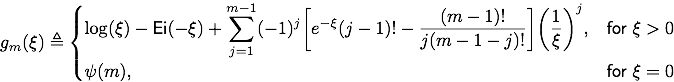for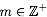. Here, the function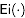denotes the exponential integral function defined as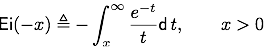and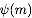is Euler's psi function given by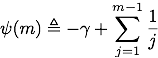where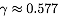denotes Euler's constant. Note that the functionsare continuous, monotonically increasing, and concave in the interval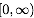for all. In particular note thatare continuous at zero for all.

## Proof

This lemma and a proof for it can be found in (Appendix X, Lemma 10.1)

 Amos Lapidoth, Stefan M. Moser: Capacity Bounds via Duality with Applications to Multiple-Antenna Systems on Flat Fading Channels, IEEE Transactions on Information Theory, vol. 49, no. 10, pp. 2426–2467, October 2003. (Abstract) [.pdf]

and in (Appendix A, Lemma A.6)

 Stefan M. Moser: Duality-Based Bounds on Channel Capacity, Ph.D. thesis, Swiss Federal Institute of Technology (ETH), Switzerland, October 2004. Under the supervision of Prof. Dr. Amos Lapidoth. Diss. ETH No. 15769. Hartung-Gorre Verlag Konstanz, January 2005, ISBN: 3-89649-956-4. (Abstract, Download)

## Remarks

• The probability density function of a noncentral chi-square distributed random variable with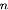degrees of freedom is given by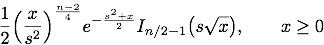where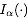denotes the modified Bessel function of the first kind of order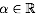. So basically the lemma gives an analytic expression for the following integral: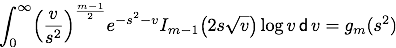Note that the real and imaginary parts of a circularly-symmetric zero-mean complex Gaussian random variable are independent zero-mean real Gaussian random variables of variance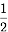.
• From this lemma it immediately follows that for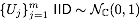with arbitrary complex constantsand a non-zero complex constant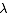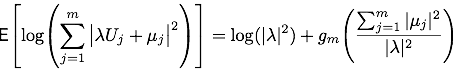• Note that a noncentrality parameter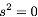leads to a central chi-square distribution (with an even number of degrees of freedom) for which the expected logarithm has been known to be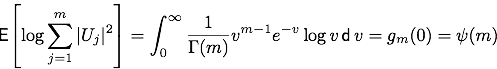See (4.352-1.) in

 I.S. Gradshteyn, I.M. Ryzhik: Table of Integrals, Series and Products, 6th edition, Academic Press, 2000. ISBN: 0-12-294757-6.

The most common situation for this special case is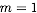where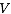consists only of the squared magnitude of one complex Gaussian random variable (a squared Rayleigh distribution or exponential distribution). In this case the expected logarithm is known to be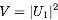.

• Note that forthe random variableis said to have a squared Rician distribution. In this case the lemma proves the expected logarithm to be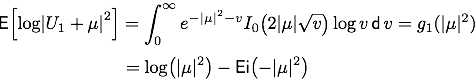• Note thatcan be bounded as follows: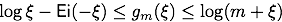For a proof see Appendix B in

 Stefan M. Moser: The Fading Number of Memoryless Multiple-Input Multiple-Output Fading Channels, IEEE Transactions on Information Theory, vol. 53, no. 7, pp. 2652–2666, July 2007. (Abstract) [.pdf]

## Keywords

Chi-square, chi-squared, noncentral chi-square, noncentral chi-squared, expected logarithm, Rayleigh, Rice, Ricean, Rician.

## Generalizations

The following lemma has been proven in (Appendix A, Lemma 3)

 Angel Lozano, Antonia M. Tulino, Sergio Verdú: High-SNR Power Offset in Multiantenna Communication, IEEE Transactions on Information Theory, vol. 51, no. 12, pp. 4134-4151, December 2005.
Lemma: Consider an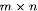random matrix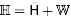with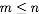, where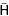is deterministic while the entries of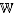are zero-mean unit-variance IID complex Gaussian. Denoting by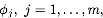the eigenvalues of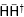we have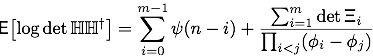where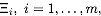is an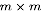matrix with entries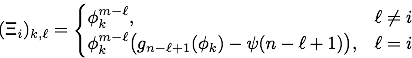-||-   _|_ _|_     /    __|__   Stefan M. Moser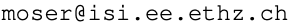[-]     --__|__   /__\    /__   Senior Scientist, ETH Zurich, Switzerland
_|_     -- --|-    _     /  /   Adjunct Professor, National Chiao Tung University, Taiwan
/ \     []  \|    |_|   / \/    Web: https://moser-isi.ethz.ch/